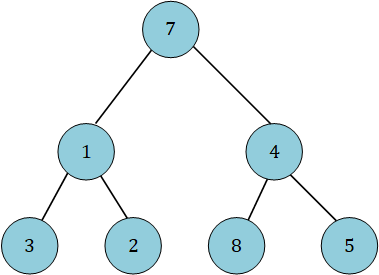New update is available. Click here to update.

# Lowest Common Ancestor of a Binary Tree III

Posted: 24 Mar, 2021
Difficulty: Moderate

## PROBLEM STATEMENT

#### Note:

``````Let ‘TREE’ be a binary tree. The lowest common ancestor of two nodes, ‘N1’ and ‘N2’, is defined as the lowest node in ‘TREE’ with ‘N1’ and ‘N2’ as descendants (where we allow a node to be a descendant of itself).
``````

#### Input Format:

``````The first line of input contains an integer 'T' representing the number of test cases.

The first line of each test case contains two single space-separated integers, ‘N1’ and ‘N2’, representing the nodes for which LCA has to be found.

The second line of each test case contains elements in the level order form. The line consists of values of nodes separated by a single space. In case a node is ‘NULL’, we take -1 in its place.
``````

#### For example:

``````The input for the tree depicted in the below image would be :
````````````7
1 4
3 2 8 5
-1 -1 -1 -1 -1 -1 -1 -1
``````

#### Explanation :

``````Level 1 :
The root node of the tree is 7

Level 2 :
Left child of 7 = 1
Right child of 7 = 4

Level 3 :
Left child of 1 = 3
Right child of 1 = 2
Left child of 4 = 8
Right child of 4 = 5

Level 4 :
Left child of 3 = NULL(-1)
Right child of 3 = NULL(-1)
Left child of 2 = NULL(-1)
Right child of 2 = NULL(-1)
Left child of 8 = NULL(-1)
Right child of 8 = NULL(-1)
Left child of 5 = NULL(-1)
Right child of 5 = NULL(-1)

The first not-null node(of the previous level) is treated as the parent of the first two nodes of the current level. The second not-null node (of the previous level) is treated as the parent node for the next two nodes of the current level and so on.
The input ends when all nodes at the last level are ‘NULL’(-1).
``````
##### Note :
``````The above format was just to provide clarity on how the input is formed for a given tree.

The sequence will be put together in a single line separated by a single space. Hence, for the above-depicted tree, the input will be given as:

7 1 4 3 2 8 5 -1 -1 -1 -1 -1 -1 -1 -1
``````
##### Output Format :
``````For each test case, print a single line containing a single integer denoting the LCA of given nodes N1 and N2.

The output for each test case is printed in a separate line.
``````

#### Note:

``````You do not need to print anything, it has already been taken care of. Just implement the given function.
``````
##### Constraints :
``````1 <= T <= 100
1 <= N <= 10 ^ 4
1 <= DATA <= 10 ^ 4
N1 != N2
N1 and N2 exist in the ‘TREE’.
The ‘TREE’ contains unique nodes.

Where ‘T’ is the number of test cases, ‘N’ is the number of nodes in the ‘TREE’, ‘DATA’ represents the value of the node, ‘N1’ and ‘N2’ represent the nodes of which LCA has to be found.

Time limit: 1 sec.
``````
SIMILAR PROBLEMS

Ninja and Tree

Posted: 5 Feb, 2022
Difficulty: Ninja

Kth Largest Element in BST

Posted: 12 Mar, 2022
Difficulty: Moderate

Height of Binary Tree

Posted: 22 Apr, 2022
Difficulty: Easy

Min Heap

Posted: 5 May, 2022
Difficulty: Moderate

Locked Binary Tree

Posted: 12 May, 2022
Difficulty: Easy